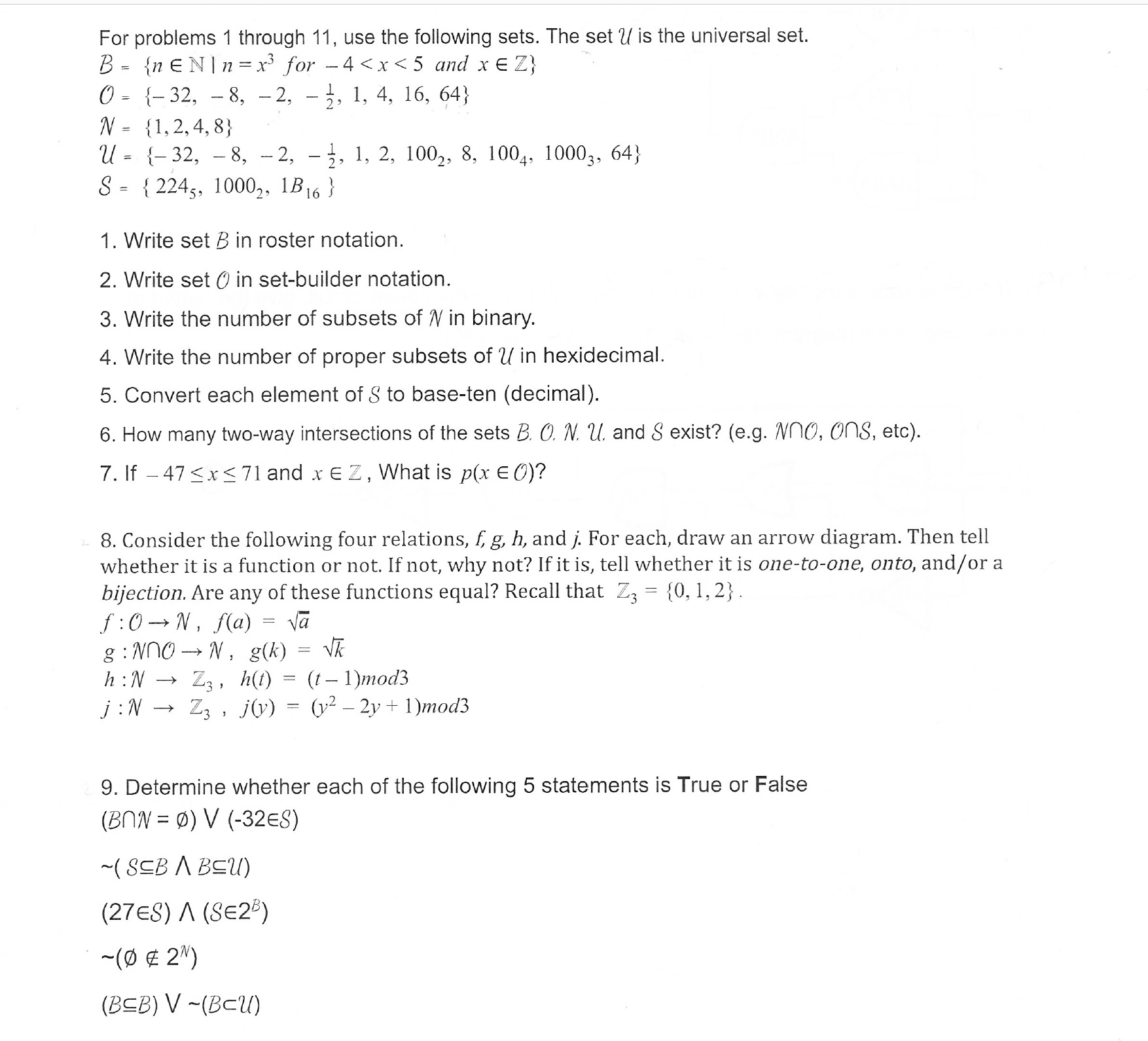# For problems 1 through 11, use the following sets. The set U is the universal set.0- -32, - 8, -2, - 3, 1, 4, 16, 64)N-1,2,4,8)U= {-32,-8,-2, .. 1, 2, 100-, 8. 1004. 10003, 64)S-2245, 1000,, B16)1. Write set B in roster notation.2. Write set O in set-builder notation3. Write the number of subsets of N in binary4. Write the number of proper subsets of U in hexidecimal.5. Convert each element of S to base-ten (decimal)6. How many two-way intersections of the sets B. O. N u. and S exist? (e.g. Nno, ons, etc)7. If -47

Question
1 viewshelp_outlineImage TranscriptioncloseFor problems 1 through 11, use the following sets. The set U is the universal set. 0- -32, - 8, -2, - 3, 1, 4, 16, 64) N-1,2,4,8) U= {-32,-8,-2, .. 1, 2, 100-, 8. 1004. 10003, 64) S-2245, 1000,, B16) 1. Write set B in roster notation. 2. Write set O in set-builder notation 3. Write the number of subsets of N in binary 4. Write the number of proper subsets of U in hexidecimal. 5. Convert each element of S to base-ten (decimal) 6. How many two-way intersections of the sets B. O. N u. and S exist? (e.g. Nno, ons, etc) 7. If -47
check_circle

Step 1

Hey, since there are multiple questions posted, we will answer first question. If you want any specific question to be answered then please submit that question only or specify the question number in your message.

Step 2

The given set is,

Step 3

The roaster notation is a list of numbers or elements separated by commas and enclo...

### Want to see the full answer?

See Solution

#### Want to see this answer and more?

Solutions are written by subject experts who are available 24/7. Questions are typically answered within 1 hour.*

See Solution
*Response times may vary by subject and question.
Tagged in

### Math